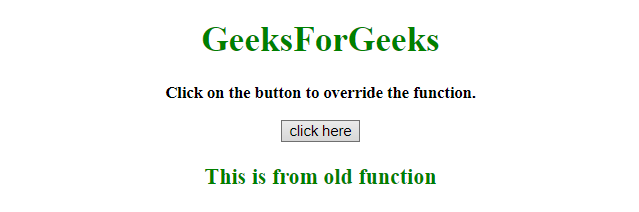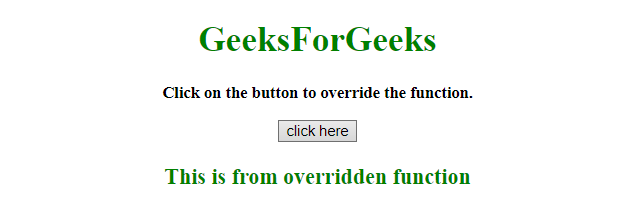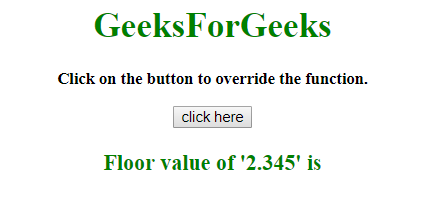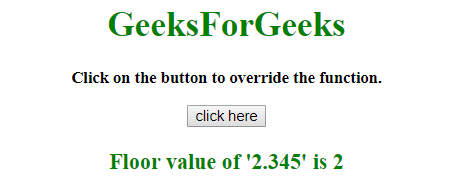# How to override a JavaScript function ?

• Last Updated : 23 Aug, 2019

Given an HTML document and the task is to override the function, either predefined function or user-defined function using JavaScript.

Approach: When we run the script then Fun() function called. After clicking the button the GFG_Fun() function called and this function contains another function which will run.

Hey geek! The constant emerging technologies in the world of web development always keeps the excitement for this subject through the roof. But before you tackle the big projects, we suggest you start by learning the basics. Kickstart your web development journey by learning JS concepts with our JavaScript Course. Now at it's lowest price ever!

Example 1: In this example, a function written by the user is override.

 `  ` `<``html``>  ` ` `  `<``head``>  ` `    ``<``title``>  ` `        ``How to override a JavaScript function ? ` `    `` ` ` ` ` `  `<``body` `style` `= ``"text-align:center;"``>  ` `     `  `    ``<``h1` `style` `= ``"color:green;"` `id` `= ``"h1"``>  ` `        ``GeeksForGeeks  ` `    `` ` `     `  `    ``<``p` `id` `= ``"GFG_UP"` `style` `= ` `        ``"font-size: 15px; font-weight: bold;"``> ` `    `` ` `     `  `    ``<``button` `onclick` `= ``"GFG_Fun()"``> ` `        ``click here ` `    `` ` `     `  `    ``<``p` `id` `= ``"GFG_DOWN"` `style` `=  ` `        ``"color:green; font-size: 20px; font-weight: bold;"``> ` `    `` ` `     `  `    ``<``script``>  ` `        ``var up = document.getElementById('GFG_UP');      ` `        ``up.innerHTML = "Click on the button to override" ` `                    ``+ " the function."; ` `         `  `        ``var down = document.getElementById('GFG_DOWN'); ` `         `  `        ``function Fun() { ` `            ``return "This is from old function"; ` `        ``} ` `         `  `        ``down.innerHTML = Fun(); ` `         `  `        ``function GFG_Fun() { ` `            ``var newFun = Fun; ` `            ``Fun = function() { ` `                ``return "This is from overridden function"; ` `            ``} ` `            ``down.innerHTML = Fun(); ` `        ``} ` `    ``  ` `  ` ` `  ` `

Output:

• Before clicking on the button:• After clicking on the button:Example 2: In this example, a parseFloat method is override.

 `  ` `<``html``>  ` ` `  `<``head``>  ` `    ``<``title``>  ` `        ``How to override a JavaScript function ? ` `    `` ` ` ` ` `  `<``body` `style` `= ``"text-align:center;"``>  ` `     `  `    ``<``h1` `style` `= ``"color:green;"` `id` `= ``"h1"``>  ` `        ``GeeksForGeeks  ` `    `` ` `     `  `    ``<``p` `id` `= ``"GFG_UP"` `style` `=  ` `        ``"font-size: 15px; font-weight: bold;"``> ` `    `` ` `         `  `    ``<``button` `onclick` `= ``"GFG_Fun()"``> ` `        ``click here ` `    `` ` `     `  `    ``<``p` `id` `= ``"GFG_DOWN"` `style` `=  ` `        ``"color:green; font-size: 20px; font-weight: bold;"``> ` `    `` ` `     `  `    ``<``script``>  ` `        ``var up = document.getElementById('GFG_UP');      ` `         `  `        ``up.innerHTML = "Click on the button to override" ` `                    ``+ " the function."; ` `         `  `        ``var down = document.getElementById('GFG_DOWN'); ` `         `  `        ``down.innerHTML = "Floor value of '2.345' is "; ` `         `  `        ``function GFG_Fun() { ` `             `  `            ``// Override ` `            ``parseFloat = function(x) { ` `                ``return "Floor value of '2.345' is " ` `                            ``+ Math.floor(x); ` `            ``} ` `             `  `            ``// Overriding the parseFloat function and ` `            ``// use it as Math.floor ` `            ``down.innerHTML = parseFloat(2.345);  ` `        ``} ` `    ``  ` `  ` ` `  ` `

Output:

• Before Click the Button:• After Click the Button:My Personal Notes arrow_drop_up
Recommended Articles
Page :